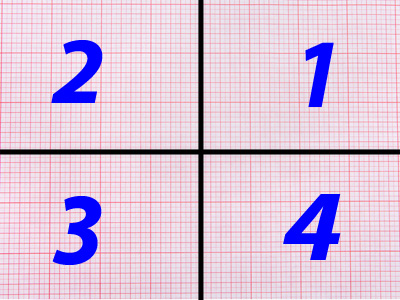There are four quadrants on a graph.

# Position 2 (Medium)

Welcome to the second of our Medium Eleven Plus maths quizzes on position. In the first we learned where coordinates lie on the x and y axes, and also which quadrant they are in. This one gives you more opportunity to practice placing them.

There are four quadrants on a graph:

• The first quadrant has positive x and y coordinates
• The second quadrant has negative x coordinates but positive y coordinates
• The third quadrant has negative x and y coordinates
• The fourth quadrant has negative x coordinates but positive y coordinates

Try to remember these if you can. They’ll make your maths tests so much easier!

Now, on with the quiz. Good luck!

1.
What shape do you get if you join up the points (0, 0), (2, 0), (1, 1) with straight lines and in which quadrant does it lie?
A triangle in the first quadrant
A triangle in the fourth quadrant
A square in the first quadrant
A square in the fourth quadrant
(0, 0) and (2, 0) lie on the coordinate axes, but (1, 1) lies in the first quadrant and this makes the triangle lie in this quadrant
2.
The third quadrant of the coordinate plane contains all the points (x, y) where both the x value and the y value are negative. Which of the following points lies in the third quadrant?
(-8, 2)
(8, 2)
(8, -2)
(-8, -2)
Both the x value and the y value are negative
3.
A square is drawn on graph paper, starting with its top-right corner on (2, 0). Which other points will the square pass through?
(2, 2), (-2, -2), (0, 0)
(2, -2), (-2, 2), (0, 0)
(2, -2), (-2, -2), (0, 2)
(2, -2), (0, -2), (0, 0)
The best way to see which points the square will go through is to draw a diagram
4.
Where does the point (-9, 0) lie on the coordinate plane?
It lies on the negative part of the y-axis to the left of the origin (0, 0)
It lies on the positive part of the y-axis to the right of the origin (0, 0)
It lies on the negative part of the x-axis to the left of the origin (0, 0)
It lies on the positive part of the x-axis to the right of the origin (0, 0)
(x, y) = (-9, 0): the y value is 0, so the point lies at -9 on the negative part of the x-axis
5.
The fourth quadrant of the coordinate plane contains all the points (x, y) where the x value is positive and the y value is negative. Which of the following points lies in the fourth quadrant?
(-4, 2)
(4, -2)
(-4, -2)
(4, 2)
The x value is positive and the y value is negative
6.
Where does the point (0, -7) lie on the coordinate plane?
It lies on the negative part of the y-axis below the origin (0, 0)
It lies on the positive part of the y-axis above the origin (0, 0)
It lies on the negative part of the x-axis below the origin (0, 0)
It lies on the positive part of the x-axis above the origin (0, 0)
(x, y) = (0, -7): the x value is 0, so the point lies at -7 on the negative part of the y-axis
7.
Line A is drawn through the points (2, -1) and (2, 3), and line B is drawn through the points (-1, 2) and (3, 2). Which of the following statements about these lines is correct?
The lines are parallel
The lines intersect at right angles
The lines intersect at acute angles
The lines intersect at obtuse angles
If you draw the lines, you will see that both lines pass through the point (2, 2) at right angles
8.
What shape do you get if you join up the points (-1, 1), (-3, 1), (-3, 3), (-1, 3) with straight lines and in which quadrant does it lie in?
A square in the third quadrant
A square in the second quadrant
An oblong in the third quadrant
An oblong in the second quadrant
(-1, 1), (-3, 1), (-3, 3) and (-1, 3) all have negative x coordinates and positive y coordinates. This means that the square lies in the second quadrant
9.
The first quadrant of the coordinate plane contains all the points (x, y) where both the x and y values are positive. Which of the following points lies in the first quadrant?
(2, -7)
(-2, 7)
(-2, -7)
(2, 7)
The x and y values are BOTH positive
10.
The second quadrant of the coordinate plane contains all the points (x, y) where the x value is negative and the y value is positive. Which of the following points lies in the second quadrant?
(6, 3)
(6, -3)
(-6, 3)
(-6, -3)
The x value is negative and the y value is positive
Author:  Frank Evans